# Class 7 语言模型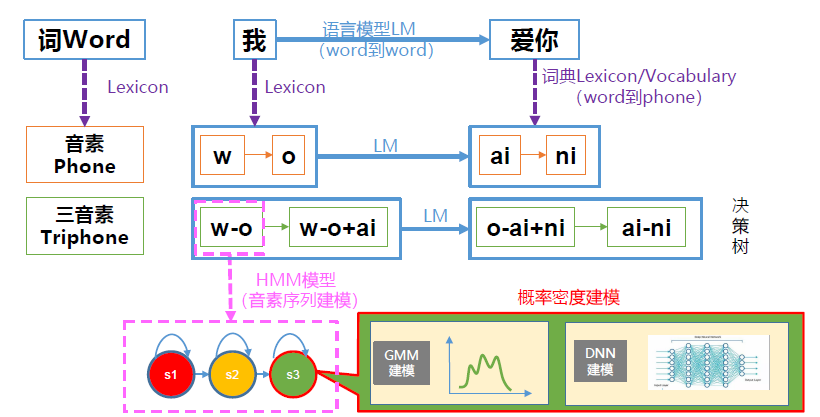#### 整体流程图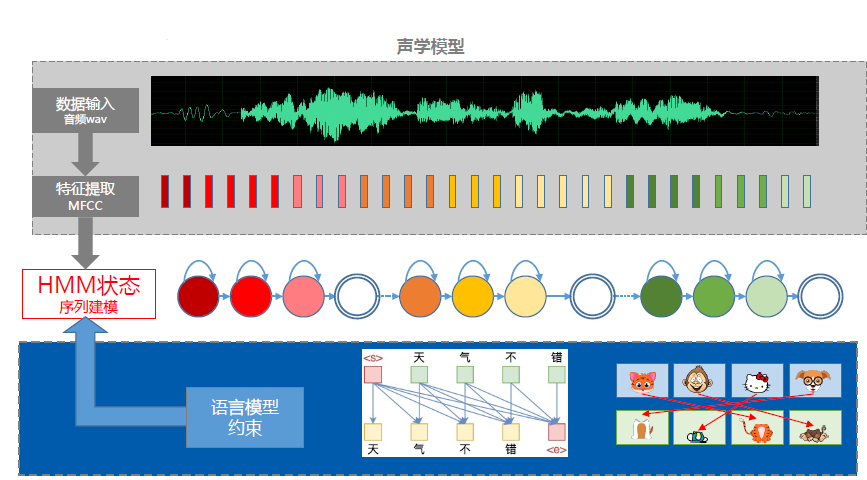### 一、统计语言模型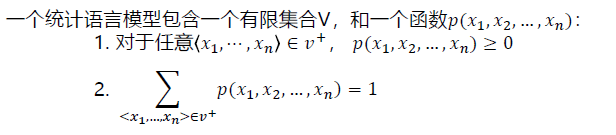1.它可以给我们任意词序列的概率，即帮助我们确定哪个词序列可能性大。
2.给定一个词序列，可以预测下一个最可能出现的词语! [用于ASR，MT等]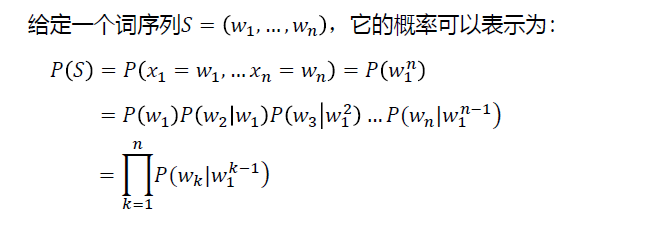• 训练集(training-set)与测试集(test-set)

• 保留集(held-out set): 从训练集中分离，用来计算一些其它参数，如插值模型中的插值系数。

• 语言模型中一些常见术语：

I uh gave a re-report yesterday

我是不是老了

• 有声停顿(fillers/filled pauses): 如uh就是一个没有实际意义的有声停顿。
• 截断(fragment):表示没有说完整，如re-。
• 词目(lemma)：词语主干(stem)相同，比如dogs和dog是一个词目。
• 词形(wordforms)：完整的词语样子，比如dogs和dog是两个词形。
• 型(type):语料库或者字典中不同单词的数目。
• 例(token):语料中单词数目。(数数)
• 字典(vocabulary)：语言模型的基本组件，规定了我对那些元素进行统计。

### 二、N-gram语言模型与评价方法

$$P(\text { buy } \mid \text { It is too expensive to })=\frac{C(\text { It is too expensive to buy })}{C(\text { It is too expensive to })}$$

N-gram：用前N-1个词作为历史，估计当前第N个词。

$$\mathrm{P}\left(\mathrm{w}{\mathrm{i}} \mid \mathrm{w}{\mathrm{i}}^{\mathrm{i}-1}\right)=\mathrm{P}\left(\mathrm{w}{\mathrm{i}} \mid \mathrm{w}{\mathrm{i}-\mathrm{N}+1}^{\mathrm{i}-1}\right)$$

$$\mathrm{P}\left(\mathrm{w}{\mathrm{i}} \mid \mathrm{w}{\mathrm{i}}^{\mathrm{i}-1}\right)=\mathrm{P}\left(\mathrm{w}{\mathrm{i}} \mid \mathrm{w}{\mathrm{i}-1}\right)$$

\begin{aligned} &\mathrm{P}\left(\mathrm{w}{\mathrm{i}} \mid \mathrm{w}{\mathrm{i}-\mathrm{N}+1}^{\mathrm{i}-1}\right)=\frac{\mathrm{C}\left(\mathrm{w}{\mathrm{i}-\mathrm{N}+1}^{\mathrm{i}-1} \mathrm{w}{\mathrm{i}}\right)}{\mathrm{C}\left(\mathrm{w}{\mathrm{i}-\mathrm{N}+1}^{\mathrm{i}-1}\right)}\ &\mathrm{P}\left(\mathrm{w} {\mathrm{i}} \mid \mathrm{w}{\mathrm{i}-1}\right)=\frac{\mathrm{C}\left(\mathrm{w}{\mathrm{i}-1} \mathrm{w}{\mathrm{i}}\right)}{\mathrm{C}\left(\mathrm{w}{\mathrm{i}-1}\right)} \end{aligned}

\begin{aligned} \mathrm{PP}(\mathrm{W}) &=\mathrm{P}\left(\mathrm{w}{1} \mathrm{w}{2} \ldots \mathrm{w}{\mathrm{N}}\right)^{-\frac{1}{N}} \ &=\sqrt{\frac{1}{\mathrm{P}\left(\mathrm{w} {1} \mathrm{w}{2} \ldots \mathrm{w}{\mathrm{N}}\right)}} \ &=\sqrt{\prod_{\mathrm{i}=1}^{\mathrm{N}} \frac{1}{\mathrm{P}\left(\mathrm{w}{\mathrm{i}} \mid \mathrm{w}{\mathrm{i}-\mathrm{N}+1}^{\mathrm{i}-1}\right)}} \end{aligned}

• PPL：考虑词数和句子数(i.e. 考虑< /s>)
• PPL1：只考虑词数

### 三、平滑算法(Smoothing)

Intuition：将每个计数都加一，从而使得任何词序列都有计数。

token: 语料中单词数目（数数）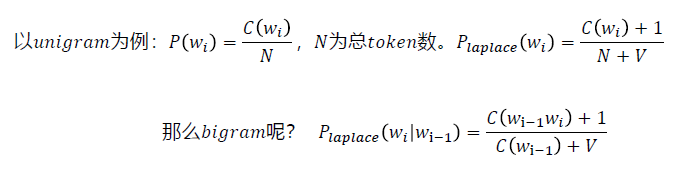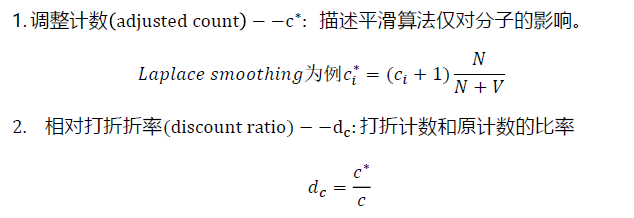Laplace Smoothing缺点：原来计数量较高的词序列，概率削减严重。

#### 古德图灵平滑(Good-turing Smoothing)

Intuition: 用你看见过一次的事件估计为看见的事件，并以此类推用看见两次的事件估计看见一次的事件…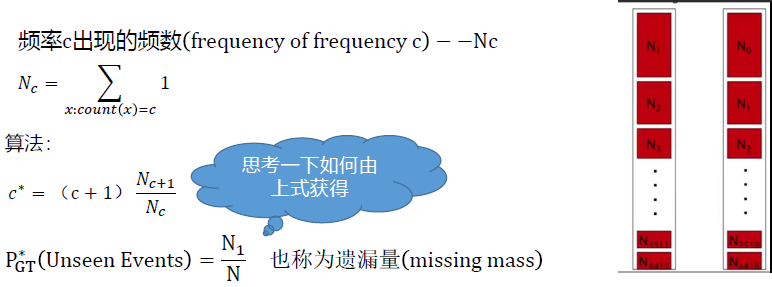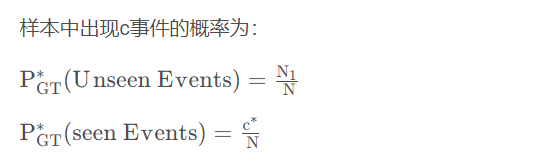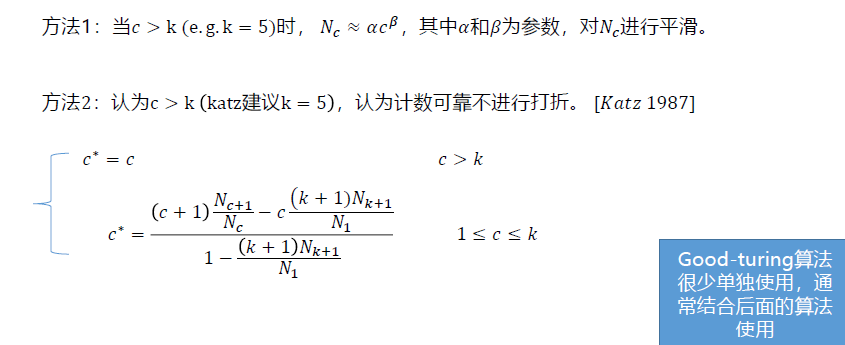#### 插值(Interpolation)平滑

Intuition：从所有N-grams估计中，把所有的概率估计混合。例如，我们优化一个tri-gram模型，我们将统计的tri-gram，bigram和unigram计数进行插值。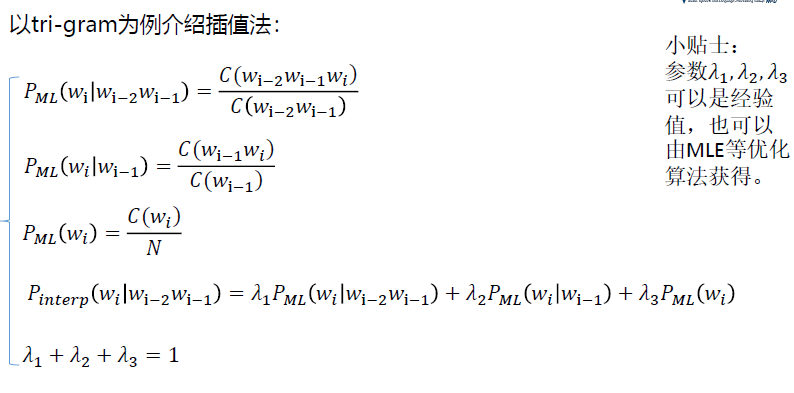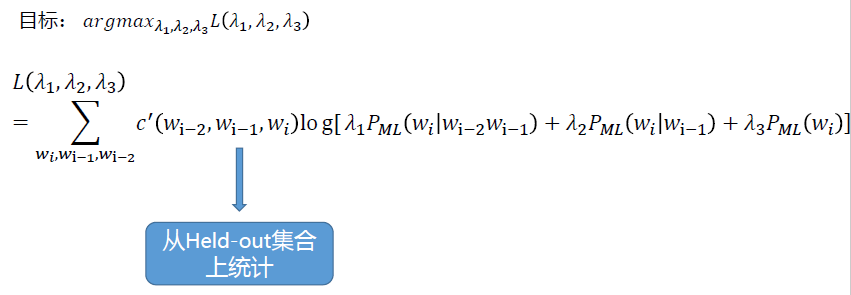#### Bucketing方法扩展Interpolation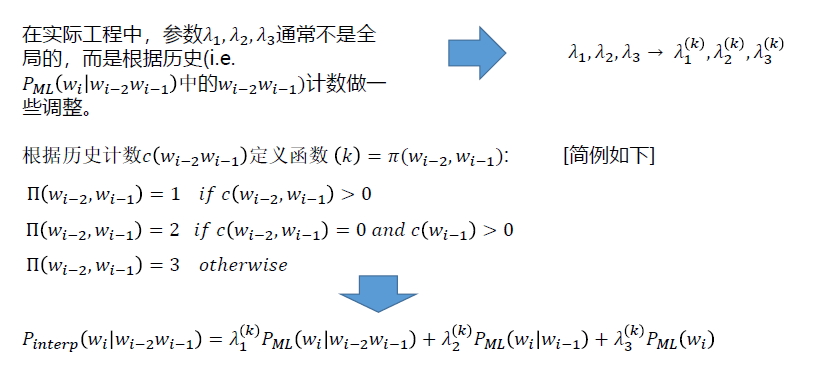#### 回退(Back off)平滑

Intuition：如果有非零的高阶语言模型，我们直接只用。只有当高级语言模型存在计数零时，我们回退到低阶语言模型。(递归)

#### 卡茨平滑(Katz Smoothing)—递归回退算法

Intuition：若N阶语言模型存在，直接使用打折后的概率(常使用Good-turing算法进行打折)；若高阶语言模型不存在(i.e. unseen events)，将打折节省出的概率量，依照N-1阶的语言模型概率进行分配，依此类推。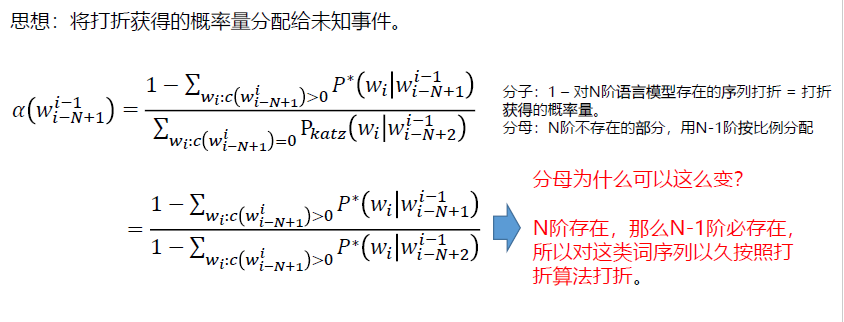#### 克奈瑟-内平滑(Kneser-Ney Smoothing)

Intuition：对于一个词，如果它在语料库中出现更多种不同上下文(context)时，它可能应该有更高的概率。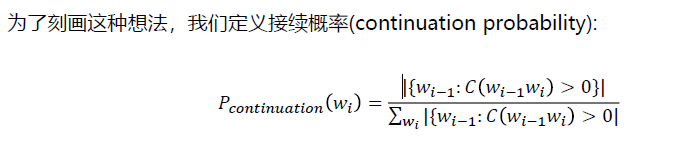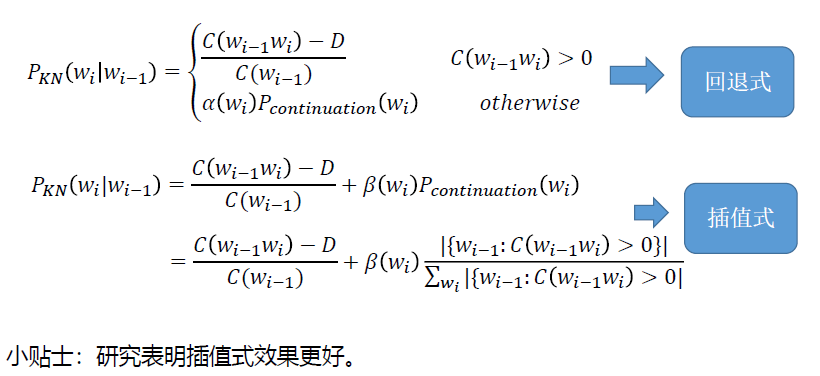### 四、语言模型的存储格式—APRA Format 及工具包

ARPA是N-gram的标准存储格式，是一个ASCII文件，小标题后边跟着一个列表，列举出所有非零的N元语法概率。每个N元语法条目中以此为：折扣后对数概率（log10格式），词序列，回退权重（log10格式）。

$$\text { e.g.: } \log {10}\left(\mathrm{w}{\mathrm{i}} \mid \mathrm{w}{\mathrm{i}-1}\right) \mathrm{w}{\mathrm{i}-1} \mathrm{w}{\mathrm{i}} \log {10} \alpha\left(\mathrm{w}{\mathrm{i}-1} \mathrm{w}{\mathrm{i}}\right)$$1.最高阶语法和 < / s > 结尾的任意阶语法没有回退权重。

2.插值模型和回退模型都可以如此储存。

#### 从代码和应用ARPA的角度思考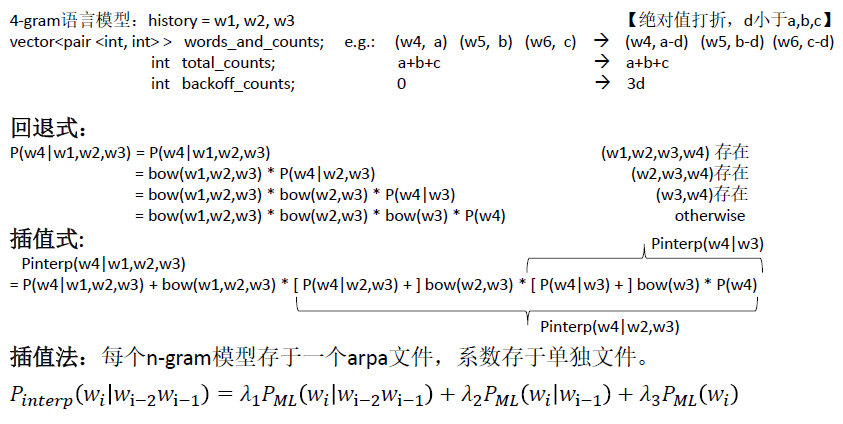### 五、RNN语言模型

\begin{aligned} P(S) &=P\left(x_{1}=w_{1}, \ldots x_{n}=w_{n}\right)=P\left(w_{1}^{n}\right) \ &=P\left(w_{1}\right) P\left(w_{2} \mid w_{1}\right) P\left(w_{3} \mid w_{1}^{2}\right) \ldots P\left(w_{n} \mid w_{1}^{n-1}\right) \ &=\prod_{k=1}^{n} P\left(w_{k} \mid w_{1}^{k-1}\right) \end{aligned}
Recurrent Neural Network正好满足这个要求。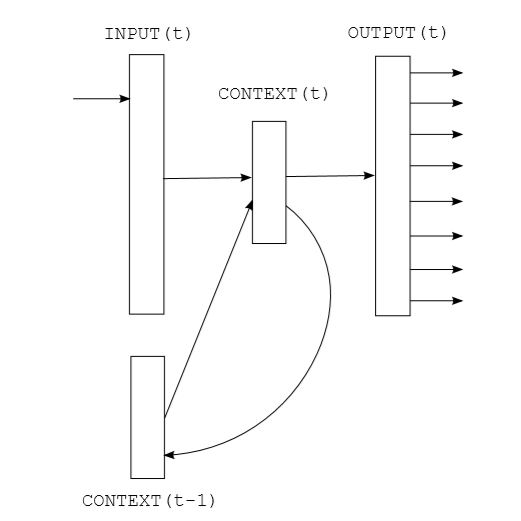Input: vocabulary size one-hot vector

Structure: Simple Recurrent Neural Network
(extend: LSTM, GRU and so on)

Output: vocabulary size vector with softmax(extend: Top N(e.g. 2k) high frequency words + 1 low frequency word bag)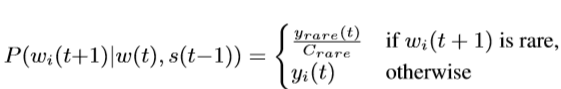### 六、其它语言模型思想简介

#### 基于类的N元语言模型(Class-based/Clustering N-gram)

Intuition:在语言学中，将具有同样语义的字词归为一类是一种常见的解决数据稀疏的方法。受此启发，在语言模型中，根据词性分析，语义分析，或者特定任务时人为设计，我们可以将词和类别建立联系，通过类别信息，帮助我们提升语言模型建模性能。（e.g.航空订票系统）

#### 缓存模型(Cache Model)

Intuition: 如果一个词在句子中用到，那么它很可能被再次用到。

### 七、大词汇量连续语音识别梳理

• 语言模型：建模word间的跳转概率。
• 字典(vocabulary)：提供word到phone的映射，及语言模型建模元素。
• HMM：建模phone或triphone等基本单元发声过程。
• GMM：建模每个HMM状态的发射概率，即声学似然分。
• 决策树：triphone等建模单元绑定(共享pdf)，解决数据稀疏问题。
• 前向后向算法：更新HMM参数。（软对齐）
• EM算法：更新GMM参数。
• Viterbi算法：解码或对齐。
• EmbeddingTraining算法：更新GMM-HMM模型参数，即ViterbiTraining。（硬对齐）
• 特征提取：从音频获取MFCC，PLP，Fbank等特征。
• DNN：建模每帧观测的后验概率，后转化为似然概率，提供给每个HMM状态。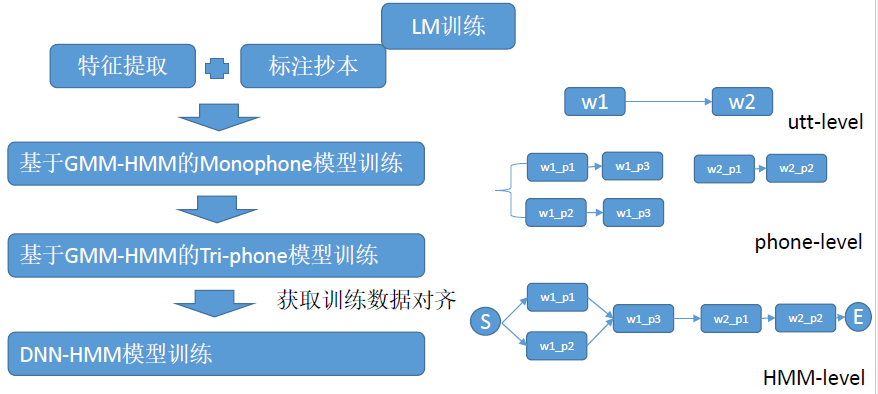## 参考

Class 7 语言模型
https://blog.baixf.tk/2022/08/17/语音识别学习/Class 7 语言模型/

2022年8月17日